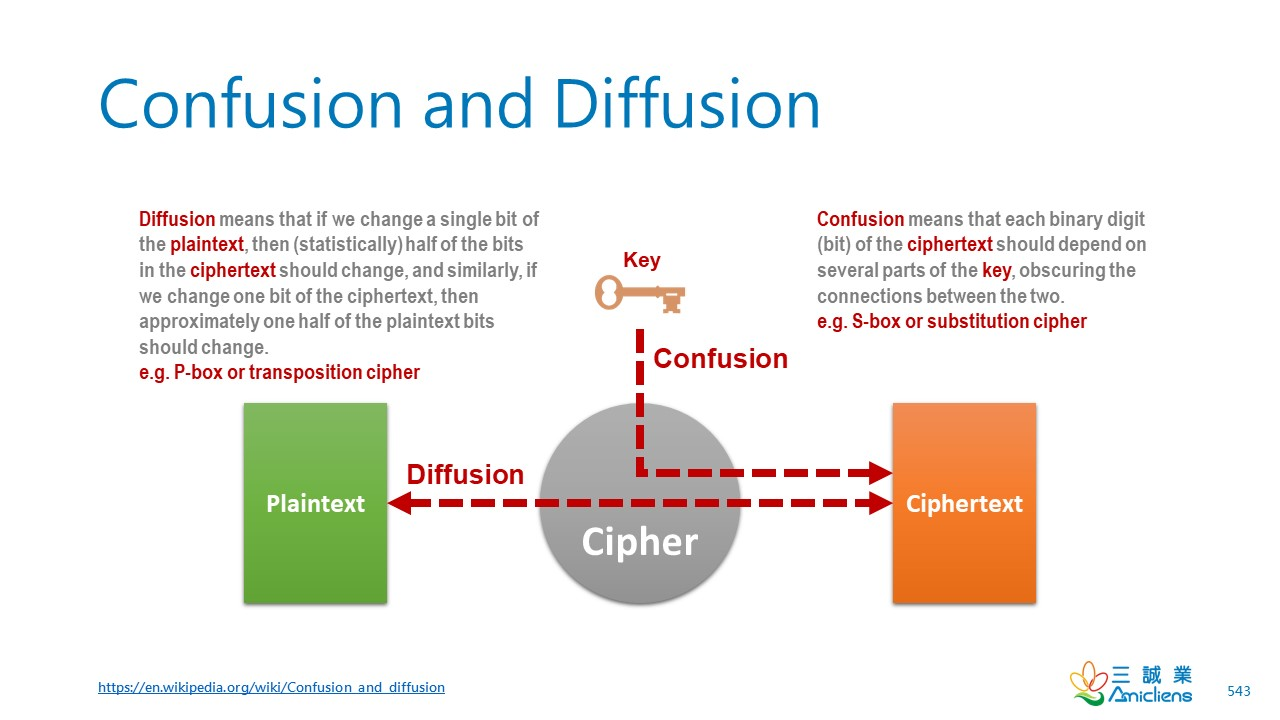# CISSP PRACTICE QUESTIONS – 20190918Confusion and diffusion are two properties of the operation of a secure cipher. Confusion refers to making the relationship between the ciphertext and the encryption key as complex and involved as possible; diffusion refers to dissipating the statistical structure of plaintext over the bulk of ciphertext. which of the following is least used in confusion and diffusion?
A. Logarithm
B. Permutation
C. Exclusive OR
D. Table lookup

Kindly be reminded that the suggested answer is for your reference only. It doesn’t matter whether you have the right or wrong answer. What really matters is your reasoning process and justifications.

My suggested answer is A. Logarithm.# Confusion and Diffusion

In cryptography, confusion and diffusion are two properties of the operation of a secure cipher identified by Claude Shannon in his 1945 classified report A Mathematical Theory of Cryptography. These properties, when present, work to thwart the application of statistics and other methods of cryptanalysis. (Wikipedia)

In Shannon’s original definitions,

• Confusion refers to making the relationship between the ciphertext and the symmetric key as complex and involved as possible.
• Diffusion refers to dissipating the statistical structure of plaintext over the bulk of ciphertext.

This complexity is generally implemented through a well-defined and repeatable series of substitutions and permutations.

• Substitution refers to the replacement of certain components (usually bits) with other components, following certain rules.
• Permutation refers to manipulation of the order of bits according to some algorithm.

## Diffusion and Avalanche Effect

In cryptography, the avalanche effect is the desirable property of cryptographic algorithms, typically block ciphers and cryptographic hash functions, wherein if an input is changed slightly (for example, flipping a single bit), the output changes significantly (e.g., half the output bits flip). In the case of high-quality block ciphers, such a small change in either the key or the plaintext should cause a drastic change in the ciphertext. The actual term was first used by Horst Feistel, although the concept dates back to at least Shannon’s diffusion. (Wikipeida)

# Table Lookup as Substitutionhttps://en.wikipedia.org/wiki/Block_cipher_mode_of_operation

## Table Lookup Example

• 00 XOR 10 = 10
• 10 turns into 11 by looking up the cipher table

# Summary

• Symmetric Cryptography is based on bitwise operations against the encryption key, while Asymmetric Cryptography relies on numerical computation (prime factorization or discrete logarithm).
• Confusion and Diffusion mostly apply to Symmetric Cryptography; both the block and stream ciphers. It depends on bitwise operations to increase complexity. e.g. XOR, modular, rotation, and the like.